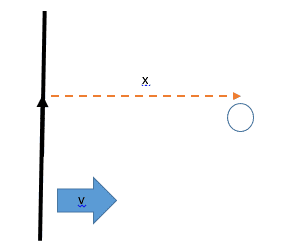# How can I derive a formula for change in B field strength?

• RobertColman
In summary, the equation for the rate of change of the strength of the magnetic field at the center of the loop is: $\frac{d}{dt}|B|=\frac{μ_{0}I}{2πV}$.

#### RobertColman

Member advised to use the homework template for posts in the homework sections of PF.
I'm a bit stuck on this question (which is homework so hints are more welcome than outright answers). The question is:

A very long wire carrying a current I is moving with speed v towards a small circular wire loop of radius r. The long wire is in the plane of the loop and is too long to be entirely shown in the diagram.The strength of a magnetic field a distance x from a long wire is
$$|B|=\frac{μ_{0}I}{2πr}$$
What is the equation for the rate of change of the strength of the magnetic field at the centre of the loop?

As a hint we are given that $$V = \frac{dx}{dt}$$

Now, I can see that the equation for the strength of the field comes from amperes law, and is essentially the magnetic field along a loop around the wire, divided by the circumference of that loop.

So it makes sense to me that given everything else in the equation is constant, delta B should come straight from the change in the circumference of a circle as the radius shrinks. Now since

$$\frac{dC}{dt}=2\pi\frac{dr}{dt}$$

and since in this case $\displaystyle{\frac{dr}{dt}}$ is simply the velocity of the wire, it seems to me that the change in the field strength at the center of the loop should simply be

$$\frac{d|B|}{dt}=\frac{μ_{0}I}{2πV}$$

however I'm getting that this answer is wrong. Can anyone explain where I've made a mistake?

RobertColman said:
however I'm getting that this answer is wrong.
Just from the dimensional analysis, one can tell that it is incorrect.
The rate of change of the strength of the B field at a fixed point is
$$\frac{d}{dt}|B|$$
Now, plug in the equation for ##|B|## into the time derivative.

•Anuran Pal
So, would you expect to get $$\frac{μ_{0}Ir^{2}-2\pi V}{2\pi r^{2}}$$ ?

RobertColman said:
So, would you expect to get $$\frac{μ_{0}Ir^{2}-2\pi V}{2\pi r^{2}}$$ ?
Is that what you get after differentiating ##|B|## with respect to ##t##?

That's what 'I' got, whether or not it is correct is what I was asking? should it be a plus instead of a minus?

as in, I used implicit differentiation to turn 1/r into -1/r^2 * dr/dt. since in this case dr/dt = -V that gives V/r^2 etc etc

ok i think i got it, i forgot that the constants I pulled out the front are being multiplied back in, so it should be $$\frac{μ_{0}IV}{2\pi r^{2}}$$?

RobertColman said:
ok i think i got it, i forgot that the constants I pulled out the front are being multiplied back in, so it should be $$\frac{μ_{0}IV}{2\pi r^{2}}$$?
Yes.

ok, Thank you so much.
I am way too stressed right now, and you were a big help :)